Note

This page is a reference documentation. It only explains the function signature, and not how to use it. Please refer to the user guide for the big picture.

# nilearn.plotting.show#

nilearn.plotting.show()[source]#

Show all the figures generated by nilearn and/or matplotlib.

This function is equivalent to `matplotlib.pyplot.show`, but is skipped on the ‘Agg’ backend where it has no effect other than to emit a warning.

## Examples using `nilearn.plotting.show`#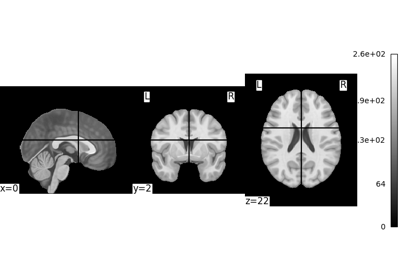Basic nilearn example: manipulating and looking at data

Basic nilearn example: manipulating and looking at data3D and 4D niimgs: handling and visualizing

3D and 4D niimgs: handling and visualizing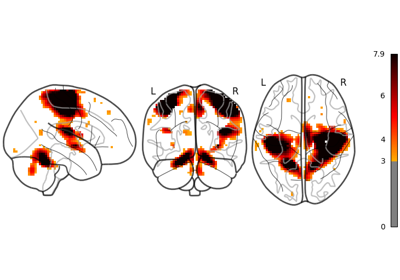Glass brain plotting in nilearn

Glass brain plotting in nilearn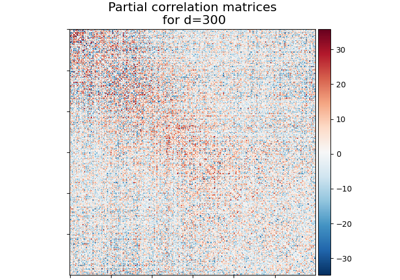Visualizing Megatrawls Network Matrices from Human Connectome Project

Visualizing Megatrawls Network Matrices from Human Connectome Project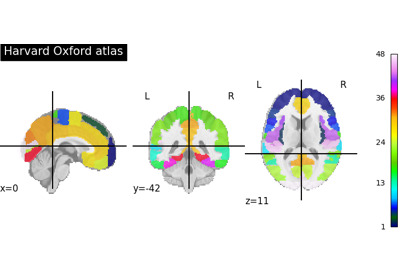Basic Atlas plotting

Basic Atlas plotting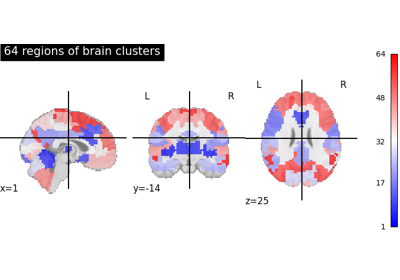Visualizing multiscale functional brain parcellations

Visualizing multiscale functional brain parcellations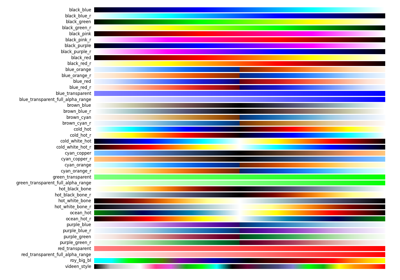Matplotlib colormaps in Nilearn

Matplotlib colormaps in Nilearn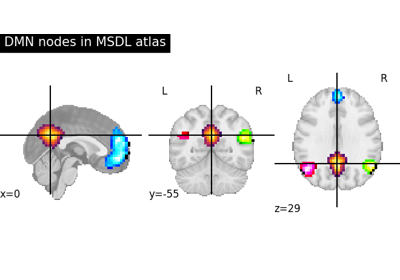Visualizing a probabilistic atlas: the default mode in the MSDL atlas

Visualizing a probabilistic atlas: the default mode in the MSDL atlasControlling the contrast of the background when plotting

Controlling the contrast of the background when plotting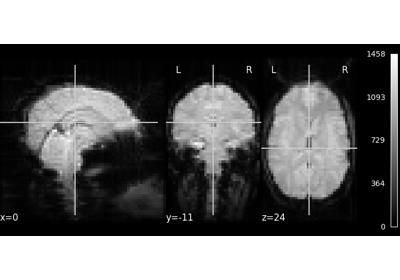NeuroImaging volumes visualization

NeuroImaging volumes visualization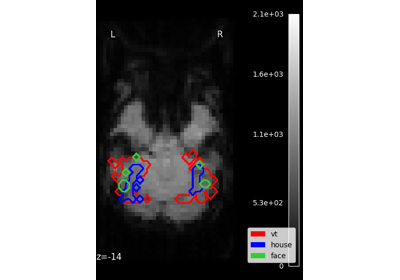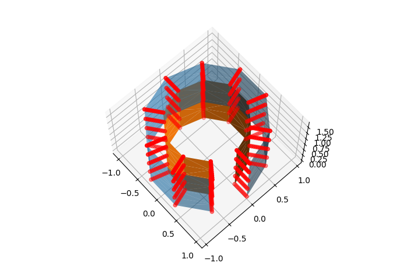Technical point: Illustration of the volume to surface sampling schemes

Technical point: Illustration of the volume to surface sampling schemes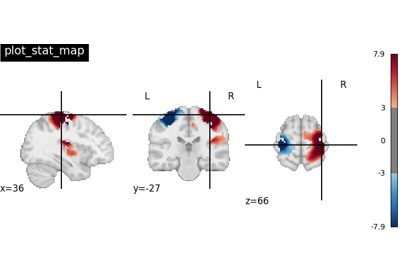Plotting tools in nilearn

Plotting tools in nilearn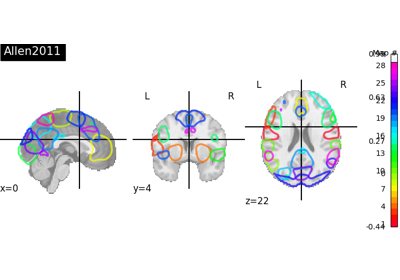Visualizing 4D probabilistic atlas maps

Visualizing 4D probabilistic atlas maps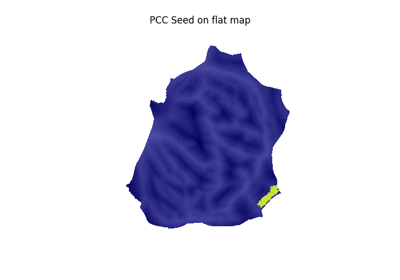Seed-based connectivity on the surface

Seed-based connectivity on the surface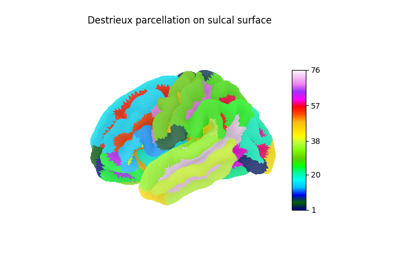Loading and plotting of a cortical surface atlas

Loading and plotting of a cortical surface atlasMaking a surface plot of a 3D statistical map

Making a surface plot of a 3D statistical mapGlass brain plotting in nilearn (all options)

Glass brain plotting in nilearn (all options)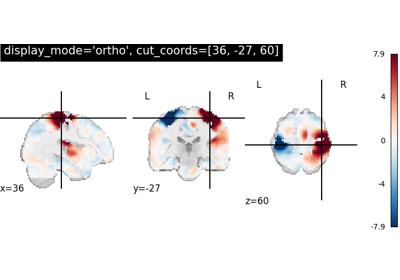More plotting tools from nilearn

More plotting tools from nilearn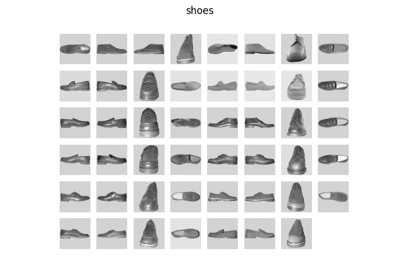Show stimuli of Haxby et al. dataset

Show stimuli of Haxby et al. dataset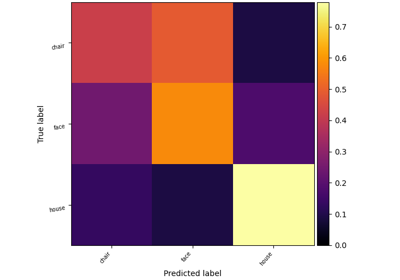Decoding with FREM: face vs house object recognition

Decoding with FREM: face vs house object recognition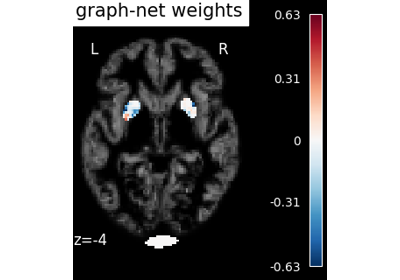Voxel-Based Morphometry on Oasis dataset with Space-Net prior

Voxel-Based Morphometry on Oasis dataset with Space-Net prior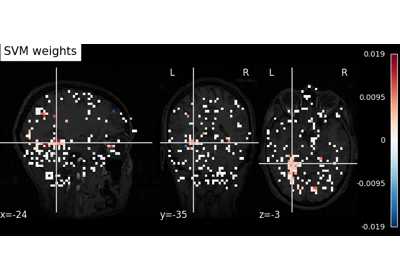Decoding with ANOVA + SVM: face vs house in the Haxby dataset

Decoding with ANOVA + SVM: face vs house in the Haxby dataset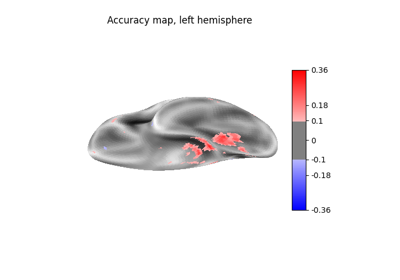Cortical surface-based searchlight decoding

Cortical surface-based searchlight decoding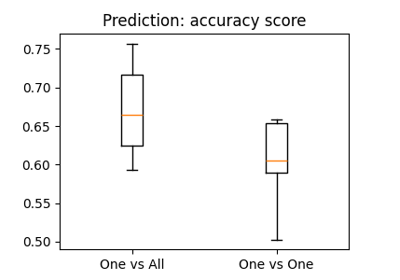The haxby dataset: different multi-class strategies

The haxby dataset: different multi-class strategiesSearchlight analysis of face vs house recognition

Searchlight analysis of face vs house recognition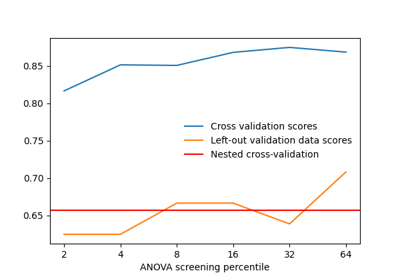Setting a parameter by cross-validation

Setting a parameter by cross-validation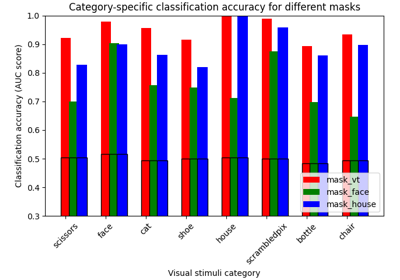ROI-based decoding analysis in Haxby et al. dataset

ROI-based decoding analysis in Haxby et al. dataset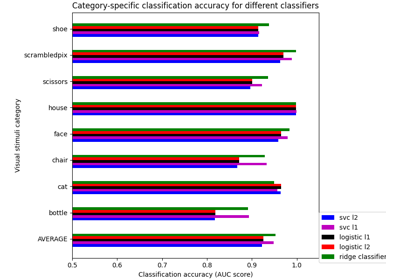Different classifiers in decoding the Haxby dataset

Different classifiers in decoding the Haxby datasetVoxel-Based Morphometry on Oasis dataset

Voxel-Based Morphometry on Oasis dataset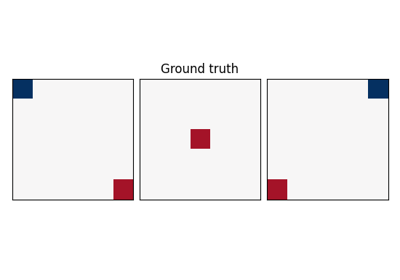Example of pattern recognition on simulated data

Example of pattern recognition on simulated data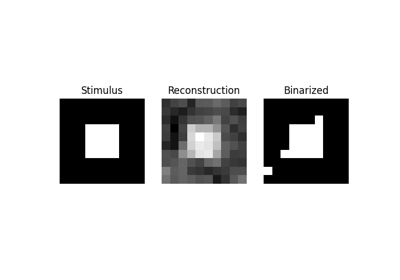Reconstruction of visual stimuli from Miyawaki et al. 2008

Reconstruction of visual stimuli from Miyawaki et al. 2008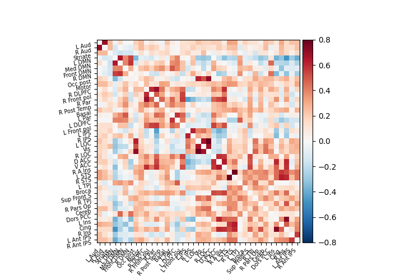Extracting signals of a probabilistic atlas of functional regions

Extracting signals of a probabilistic atlas of functional regionsComputing a connectome with sparse inverse covariance

Computing a connectome with sparse inverse covarianceConnectivity structure estimation on simulated data

Connectivity structure estimation on simulated data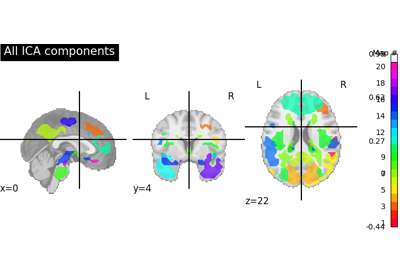Deriving spatial maps from group fMRI data using ICA and Dictionary Learning

Deriving spatial maps from group fMRI data using ICA and Dictionary Learning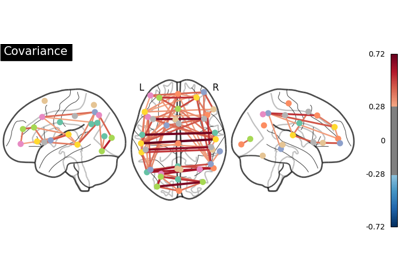Group Sparse inverse covariance for multi-subject connectome

Group Sparse inverse covariance for multi-subject connectome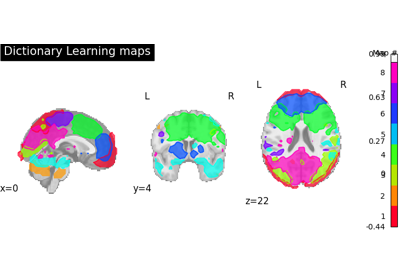Regions extraction using dictionary learning and functional connectomes

Regions extraction using dictionary learning and functional connectomes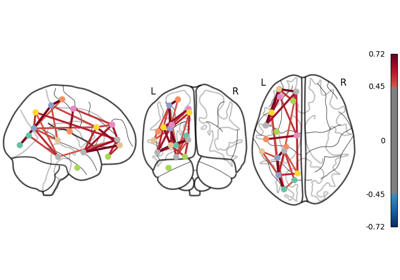Comparing connectomes on different reference atlases

Comparing connectomes on different reference atlases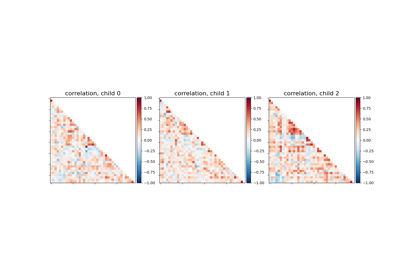Classification of age groups using functional connectivity

Classification of age groups using functional connectivity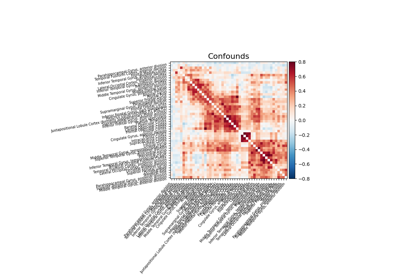Extracting signals from a brain parcellation

Extracting signals from a brain parcellation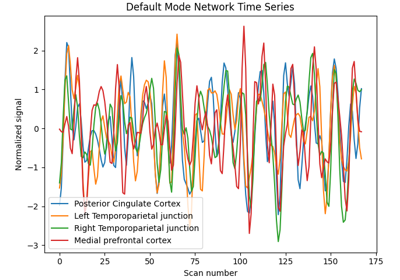Extract signals on spheres and plot a connectome

Extract signals on spheres and plot a connectomeExample of explicit fixed effects fMRI model fitting

Example of explicit fixed effects fMRI model fittingSingle-subject data (two sessions) in native space

Single-subject data (two sessions) in native spaceSimple example of two-session fMRI model fitting

Simple example of two-session fMRI model fittingExample of surface-based first-level analysis

Example of surface-based first-level analysis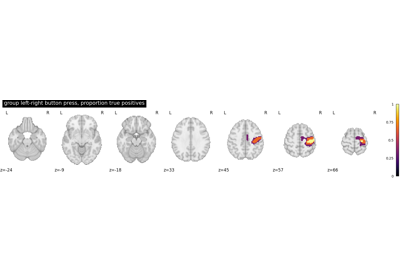Second-level fMRI model: true positive proportion in clusters

Second-level fMRI model: true positive proportion in clusters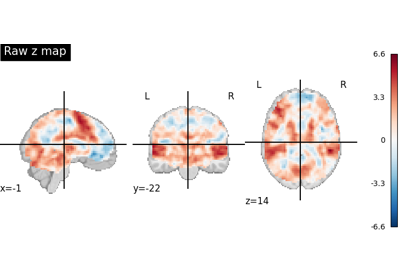Statistical testing of a second-level analysis

Statistical testing of a second-level analysis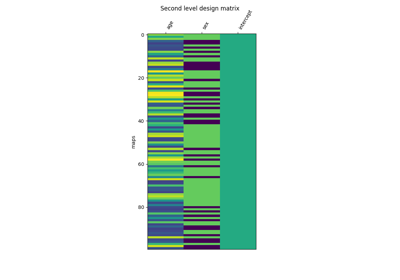Voxel-Based Morphometry on OASIS dataset

Voxel-Based Morphometry on OASIS dataset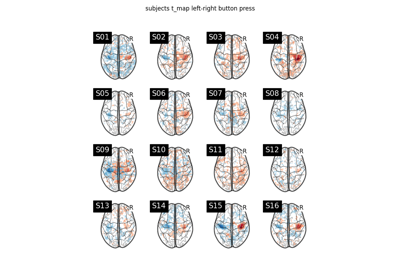Second-level fMRI model: one sample test

Second-level fMRI model: one sample test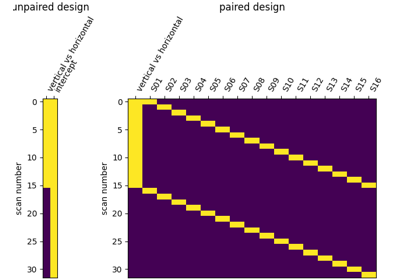Second-level fMRI model: two-sample test, unpaired and paired

Second-level fMRI model: two-sample test, unpaired and pairedExample of generic design in second-level models

Example of generic design in second-level models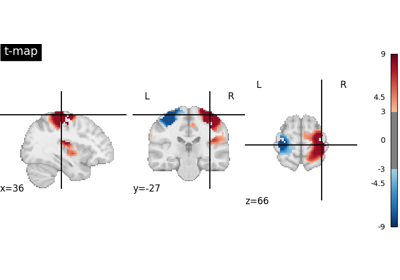Negating an image with math_img

Negating an image with math_img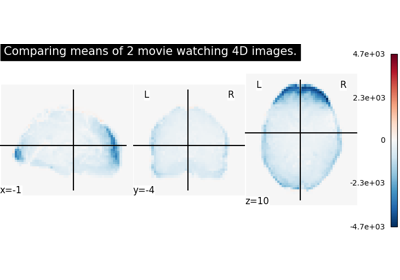Comparing the means of 2 images

Comparing the means of 2 images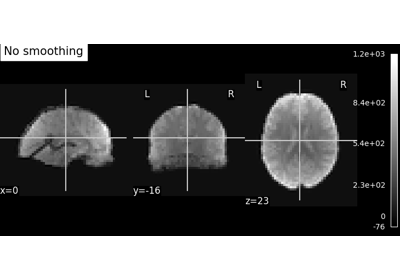Smoothing an image

Smoothing an image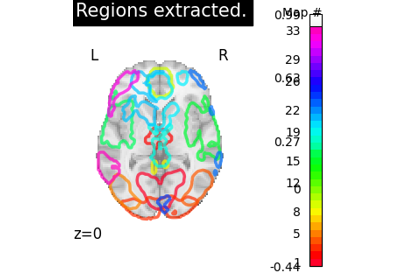Regions Extraction of Default Mode Networks using Smith Atlas

Regions Extraction of Default Mode Networks using Smith Atlas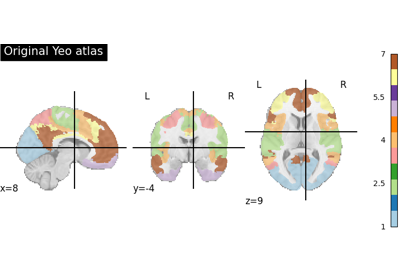Breaking an atlas of labels in separated regions

Breaking an atlas of labels in separated regions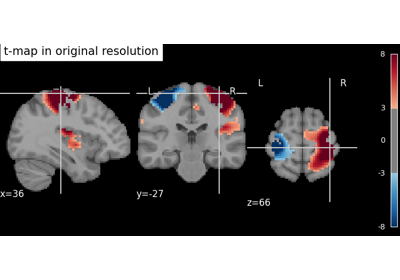Resample an image to a template

Resample an image to a template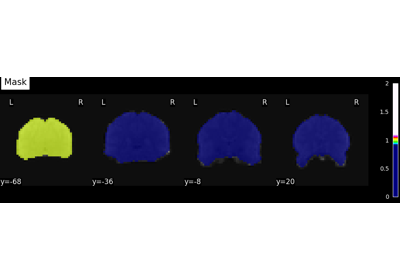Simple example of NiftiMasker use

Simple example of NiftiMasker useRegion Extraction using a t-statistical map (3D)

Region Extraction using a t-statistical map (3D)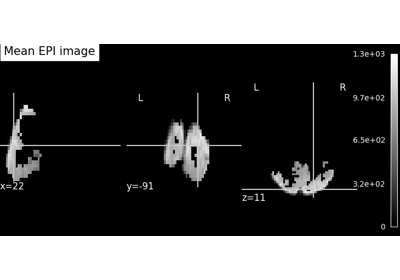Visualization of affine resamplings

Visualization of affine resamplings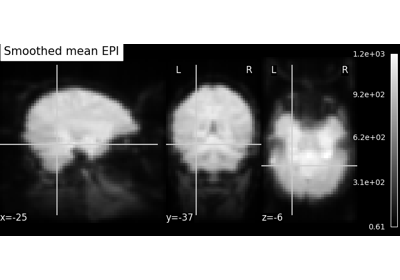Computing a Region of Interest (ROI) mask manually

Computing a Region of Interest (ROI) mask manually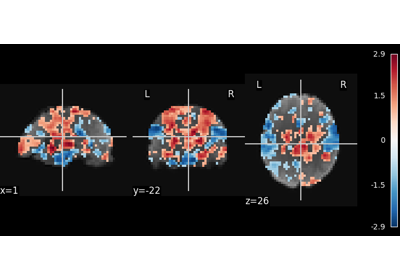Multivariate decompositions: Independent component analysis of fMRI

Multivariate decompositions: Independent component analysis of fMRIMassively univariate analysis of a calculation task from the Localizer dataset

Massively univariate analysis of a calculation task from the Localizer dataset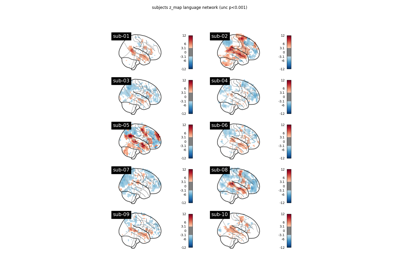BIDS dataset first and second level analysis

BIDS dataset first and second level analysis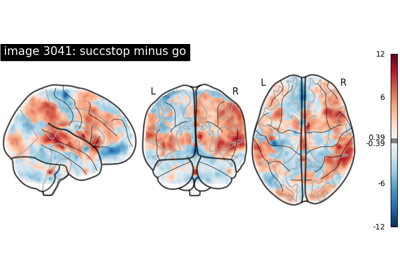NeuroVault meta-analysis of stop-go paradigm studies.

NeuroVault meta-analysis of stop-go paradigm studies.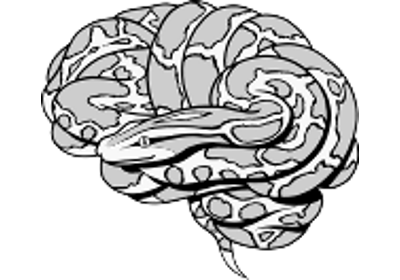Surface-based dataset first and second level analysis of a dataset

Surface-based dataset first and second level analysis of a dataset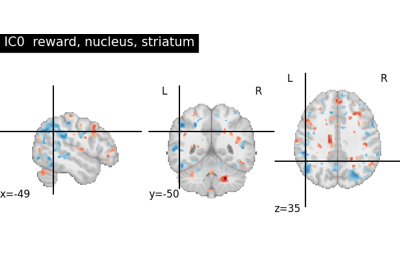NeuroVault cross-study ICA maps.

NeuroVault cross-study ICA maps.Massively univariate analysis of face vs house recognition

Massively univariate analysis of face vs house recognition